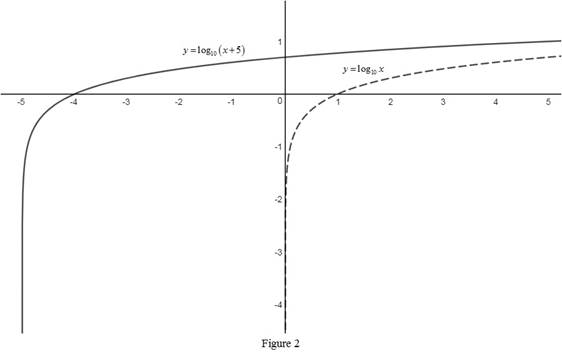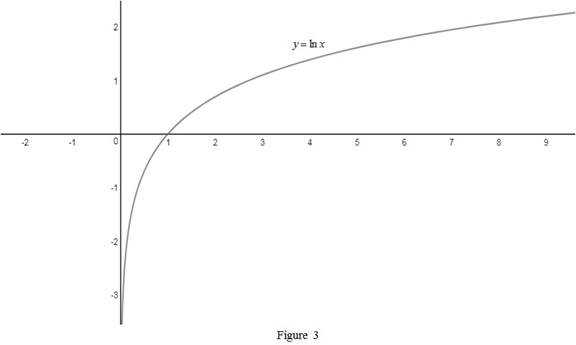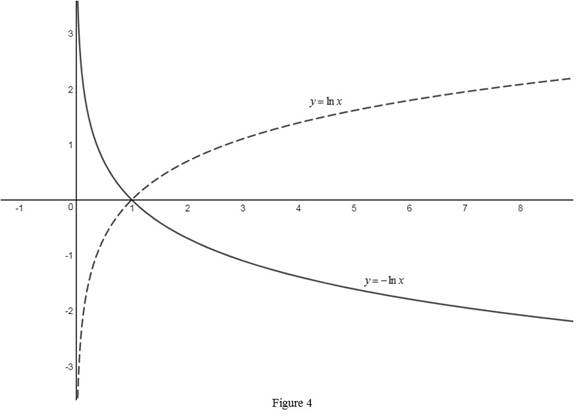# The function y = log 10 ( x + 5 ) using the graphs from Figure 12 and Figure 13; use the concept of transformations if needed.### Single Variable Calculus: Concepts...

4th Edition
James Stewart
Publisher: Cengage Learning
ISBN: 9781337687805### Single Variable Calculus: Concepts...

4th Edition
James Stewart
Publisher: Cengage Learning
ISBN: 9781337687805

#### Solutions

Chapter 1.6, Problem 47E

(a)

To determine

## To sketch: The function y=log10(x+5) using the graphs from Figure 12 and Figure 13; use the concept of transformations if needed.

Expert Solution

### Explanation of Solution

From Figure 12, the graph of the function y=log10x is shown below in Figure 1.Then, draw the graph of y=log10(x+5) , by shifting the graph y=log10x five units to the left. Thus, the graph of y=log10(x+5) is shown below in Figure 2.Observe that Figure 2 is obtained from Figure 1 in such a way that it is shifted 5 units to the left.

(b)

To determine

Expert Solution

### Explanation of Solution

From Figure 13, the graph of the function y=lnx is shown below in Figure 3.Then, draw the graph of y=lnx , by reflecting the graph y=lnx about x-axis. Thus, the graph of y=lnx is shown below in Figure 4.Observe that Figure 4 is obtained from Figure 3 in such a way that it is reflected about the x-axis.

### Have a homework question?

Subscribe to bartleby learn! Ask subject matter experts 30 homework questions each month. Plus, you’ll have access to millions of step-by-step textbook answers!